# Qlik Design Blog

All about product and Qlik solutions: scripting, data modeling, visual design, extensions, best practices, etc.

Announcements
QlikWorld Online 2021, May 10-12: Our Free, Virtual, Global Event REGISTER TODAYEmployee

## Recipe for a Pareto Analysis – Revisited

“Which products contribute to the first 80% of our turnover?”

This type of question is common in all types of business intelligence. I say “type of question” since it appears in many different forms: Sometimes it concerns products, but it can just as well concern any dimension, e.g. customer, supplier, sales person, etc. Further, here the question was about turnover, but it can just as well be e.g. number of support cases, or number of defect deliveries, etc.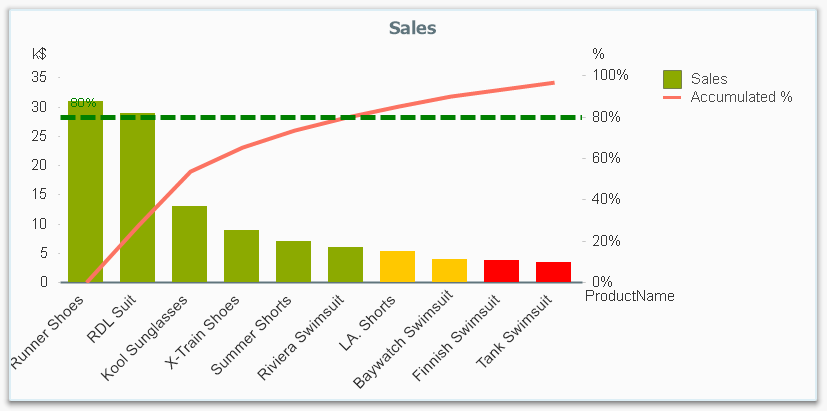It is called Pareto analysis or ABC analysis and I have already written a blog post on this topic. However, in the previous post I only explained how to create a measure which showed the Pareto class. I never showed how to create a dimension based on a Pareto classification – simply because it wasn’t possible.

But now it is.

But first things first. The logic for a Pareto analysis is that you first sort the products according to their sales numbers, then accumulate the numbers, and finally calculate the accumulated measure as a percentage of the total. The products contributing to the first 80% are your best, your “A” products. The next 10% are your “B” products, and the last 10% are your “C” products. In the above graph, these classes are shown as colors on the bars.

The previous post shows how this can be done in a chart measure using the Above() function. However, if you use the same logic, but instead inside a sorted Aggr() function, you can achieve the same thing without relying on the chart sort order. The sorted Aggr() function is a fairly recent innovation, and you can read more about it here.

The sorting is needed to calculate the proper accumulated percentages, which will give you the Pareto classes. So if you want to classify your products, the new expression to use is

=Aggr(
If(Rangesum(Above(Sum({1} Sales)/Sum({1} total Sales),1,RowNo()))<0.8, 'A',
If(Rangesum(Above(Sum({1} Sales)/Sum({1} total Sales),1,RowNo()))<0.9, 'B',
'C')),
(Product,(=Sum({1} Sales),Desc))
)

The first parameter of the Aggr() – the nested If()-functions – is in principle the same as the measure in the previous post. Look there for an explanation.

The second parameter of the Aggr(), the inner dimension, contains the magic of the sorted Aggr():

(Product,(=Sum({1} Sales),Desc))

This structured parameter specifies that the field Product should be used as dimension, and its values should be sorted descending according to Sum({1} Sales). Note the equals sign. This is necessary if you want to sort by expression.

So the Products inside the Aggr() will be sorted descending, and for each Product the accumulated relative sales in percent will be calculated, which in turn is used to determine the Pareto classes.

The set analysis {1} is necessary if you want the classification to be independent of the made selection. Without it, the classification will change every time the selection changes. But perhaps a better alternative is to use {\$<Product=>}. Then a selection in Product (or in the Pareto class itself) will not affect the classification, but all other selections will.

The expression can be used either as dimension in a chart, or in a list box. Below I have used the Pareto class as first dimension in a pivot table.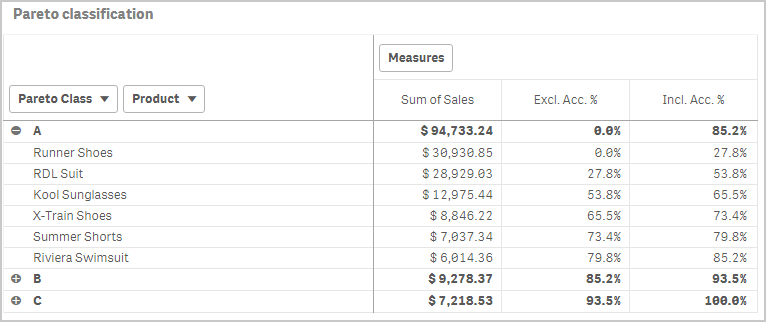If you use this expression in a list box, you can directly select the Pareto class you want to look at.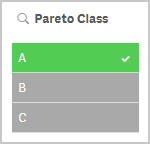The other measures in the pivot table are the exclusive and inclusive accumulated relative sales, respectively. I.e. the lower and upper bounds of the product sales share:

Exclusive accumulated relative sales (lower bound):

=Min(Aggr(
Rangesum(Above(Sum({1} Sales)/Sum({1} total Sales),1,RowNo())),
(Product,(=Sum({1} Sales),Desc))
))

Inclusive accumulated relative sales (upper bound):

=Max(Aggr(
Rangesum(Above(Sum({1} Sales)/Sum({1} total Sales),0,RowNo())),
(Product,(=Sum({1} Sales),Desc))
))

Good luck in creating your Pareto dimension!

HIC

Further reading related to this topic:

The sortable Aggr function is finally here!

Recipe for a Pareto Analysis

Recipe for an ABC Analysis

66 CommentsPartner

Hello.
You can create a pivot straight table with customer_id as a dimension.
Set sorting by expression Sum({1} Sales) Descending.
Create a measure with label "Cumulative %" and expression
RangeSum(above([Cumulative %]), Sum({1} Sales)/Sum({1} total Sales) ).
Create a measure with expression
If(Rangesum(Above([Cumulative %]))<0.8, 'A',
If(Rangesum(Above([Cumulative %]))<0.9, 'B',
'C')).

I discovered that pivot table has limitations working with interrecord functions, but straight table hasn't.

1,151 ViewsContributor II

@abeletsky thanks for suggestion. I did it but something strange happen. It looks like that in the middle of "Cumulative %" column it is restarting RangeSum (see image below). Do you have and idea what could be wrong?

I expect that last row should 100 % in "Cumulative %" column, right?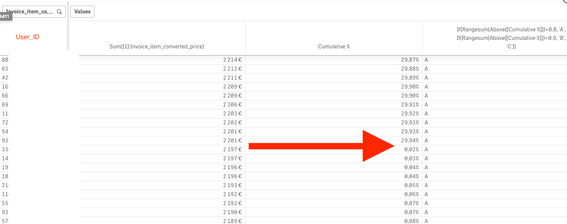1,139 ViewsPartner

Hi.

My guess you have not one dimension, so "Cumulative %"  restarts, when above() sees new dimension segment.

Try to add TOTAL in above().

1,130 ViewsPartner

Hi again.

I think I know why my proposal doesn't work properly.

It is related to "Self-referencing expression".

Quote from Qlik Sense November 2018 help: "Self-referencing expression definitions can only reliably be made in tables with fewer than 100 rows, but this may vary depending on the hardware that the Qlik engine is running on."

Link to help:

https://help.qlik.com/en-US/sense/November2018/Subsystems/Hub/Content/Sense_Hub/ChartFunctions/Inter...

1,118 Views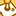Contributor

ignore

1,042 ViewsContributor

Hello,

Only a some questions:

Option #1

1) How can I make the filter of Pareto Class fixed? If I choose A for example it recalculates all over again.

Filter:  =Aggr(
If(Rangesum(Above(Sum({1} Sales)/Sum({1} total Sales),1,RowNo()))<0.5, 'A',
If(Rangesum(Above(Sum({1} Sales)/Sum({1} total Sales),1,RowNo()))<0.8, 'B',
'C')),
([Assortment Group],(=Sum({1} Sales),Desc))
)

Dimension: [Assortment Group]

Measure X: Sum(Sales)

Measure Y (Inclusive Percentage): RangeSum(Above(Sum(Sales), 0, RowNo())) / Sum(total Sales)

2) How can the Y Line does not change the colour?

The Colour Expression that I created was:

If(RangeSum(Above(Sum(Sales),1,RowNo())) / Sum(total Sales) <= 0.5, Green(),
If(RangeSum(Above(Sum(Sales),1,RowNo())) / Sum(total Sales) <= 0.8, Yellow(), ))

Option #2

Filter:  =Aggr(
If(Rangesum(Above(Sum({1} Sales)/Sum({1} total Sales),1,RowNo()))<0.5, 'A',
If(Rangesum(Above(Sum({1} Sales)/Sum({1} total Sales),1,RowNo()))<0.8, 'B',
'C')),
([Assortment Group],(=Sum({1} Sales),Desc))
)

Dimension: [Assortment Group]

Measure X: Sum(Sales)

Measure Y (Inclusive Percentage): RangeSum(Above(Sum(Sales), 0, RowNo())) / Sum(total Sales)

The Colour Expression that I created was:

=Aggr(If(RangeSum(Above(Sum(Sales), 0, RowNo())) / Sum(total Sales))<0.5,Green(),
If(RangeSum(Above(Sum(Sales), 0, RowNo())) / Sum(total Sales)<0.9, Yellow(),Red(),
([Assortment Group],(=Sum({1} Sales),Desc))))

1,038 Views